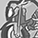Announcements
Sept. 27, 10AM ET Do more with Qlik, Insight Advisor – our intelligent AI-assistant in Qlik Sense: Register
cancel
Showing results for
Did you mean:Contributor II

## Sum with conditions.

Hi all,

I'm new to this forum so apologies in advance if I've posted this question in the wrong place.

I'm trying to create a formula where I add up the vAggMeasure only if CC_Quartile is equal to either 1,2,3 or 4. This is the formula I have so far:

Sum(TOTAL <[ProjectName]> aggr({<CC_Quartile='={1,2,3,4}'>} \$(vAggMeasure), [ProjectName], [FundName]))

However, the total which is computed using this formula includes instances where 'CC_Quartile' is a blank ('-' in QS).

Does anyone know how I can fix this?

Labels (1)
• ### SaaS

1 Solution

Accepted SolutionsSpecialist

hi,

Sum(TOTAL <[ProjectName]> aggr({<CC_Quartile='={1,2,3,4}'>} \$(vAggMeasure), [ProjectName], [FundName]))

try this
(Sum({\$<CC_Quartile = {'1','2','3','4'}>} Total AggMeasure))

ksrinivasan.

4 RepliesEmployee

Try this:

Sum(TOTAL <[ProjectName]> aggr({<CC_Quartile={1,2,3,4}>} \$(vAggMeasure), [ProjectName], [FundName]))Contributor II
Author
Thanks for coming back so quickly. Unfortunately, I’ve tried this formula and am still having the same issue.Specialist

hi,

Sum(TOTAL <[ProjectName]> aggr({<CC_Quartile='={1,2,3,4}'>} \$(vAggMeasure), [ProjectName], [FundName]))

try this
(Sum({\$<CC_Quartile = {'1','2','3','4'}>} Total AggMeasure))

ksrinivasan.Contributor II
Author

Thanks all.Tags
Community Browser